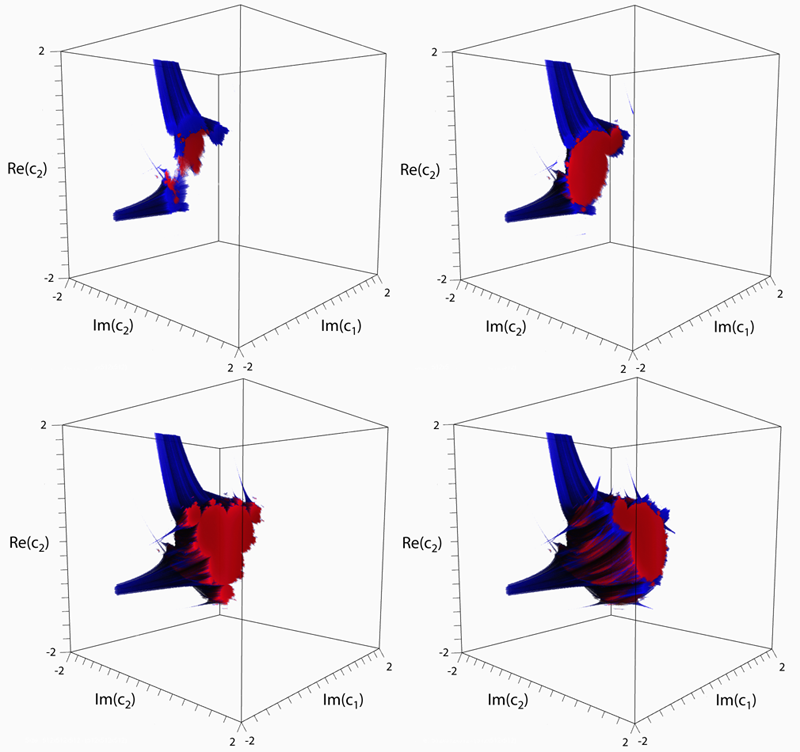# Bedouin Fractal

Created by Paul Bourke
Attributed to Russell Walsmith
April 2016

This is created by considering each point within some region of 3D space (x0,y0,z0) and evaluating the following series. The point (voxel in computer graphics language) is then assigned a scalar quantity depending on how quickly the series tends to infinity.

xn+1 = a + sin(x0)
yn+1 = b + sin(y0)
zn+1 = c + sin(z0)

where

a = xn2 - yn2 - zn2
b = 2 xn zn
c = 2 xn yn

Rendering here done using custom code to create the volumes and then the Drishti volume rendering software for the final graphics. The rendering technique is traditional volume rendering which includes assignment of both colour and opacity dependent on the voxel scalar quantity and also the estimate of local gradient to create a surface for the a lighting model.The range for which the interesting part of the model exists is -1.5 to 1.5 on all three axes. The first three renders here sample that volume 1024 times on each axis between the bounds above, so approximately 340 samples per unit distance.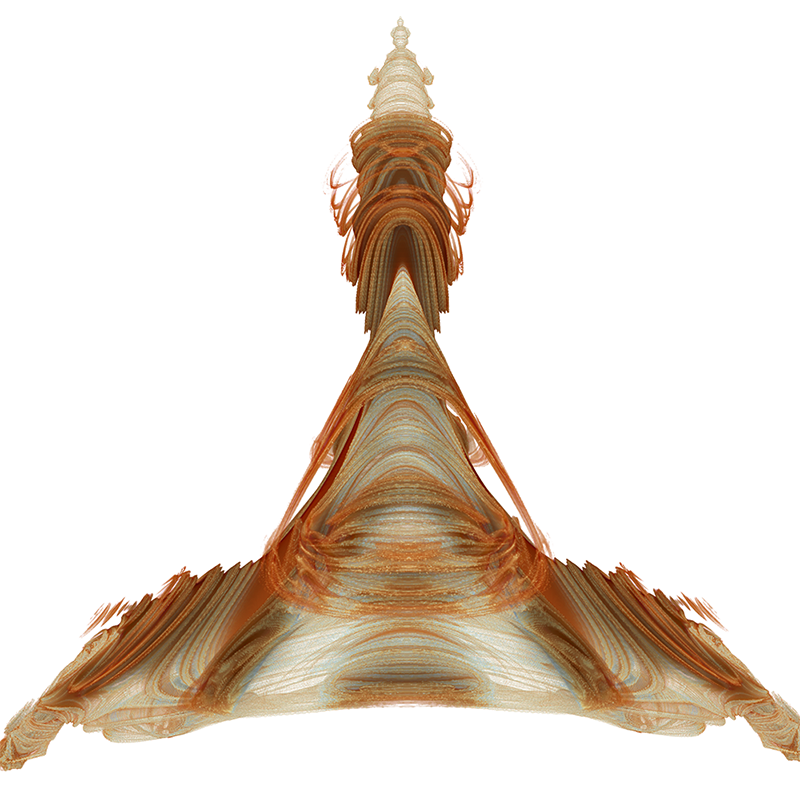The next 2 images are a further 3 times zoom factor, namely 1000 sample per unit distance.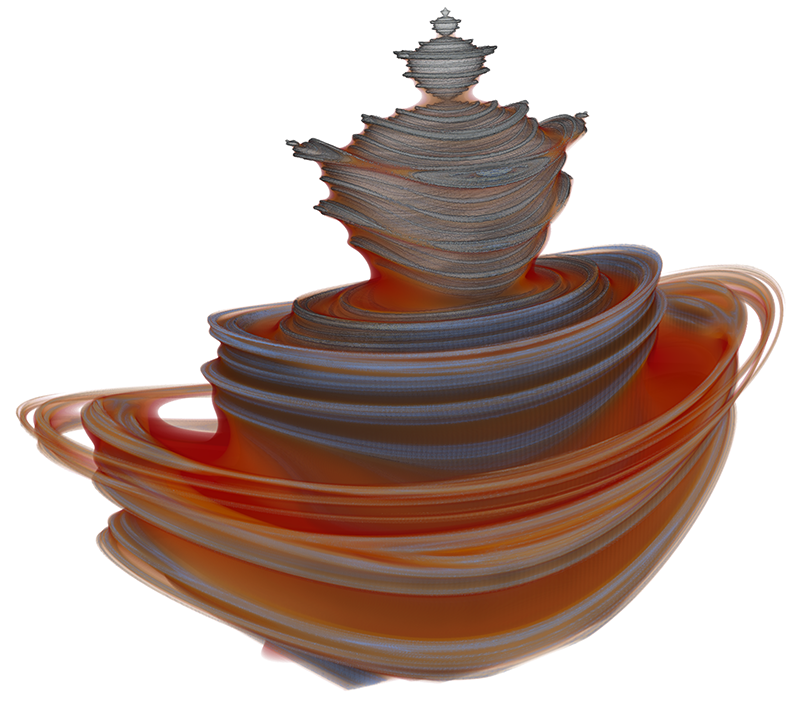# Real number MSet

Attributed to Russell Walsmith
Written by Paul Bourke
November 2006

 ```int Eval(double cx,double cy,double cz,int nmax) { int n = 0; double x1 = 0,y1 = 0,z1 = 0; double x2,y2,z2,zz = 0; while (zz < 8 && n < nmax) { x2 = x1 * x1 - y1 * y1 - z1 * z1 + cx; y2 = 2 * x1 * y1 + cy; z2 = 2 * x1 * z1 + cz; x1 = x2; y1 = y2; z1 = z2; n++; zz = x1 * x1 + y1 * y1 + z1 * z1; } return(n); } ```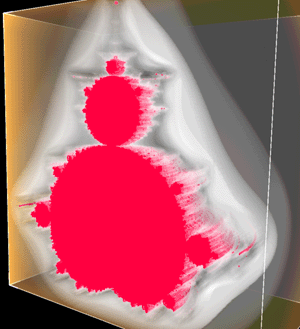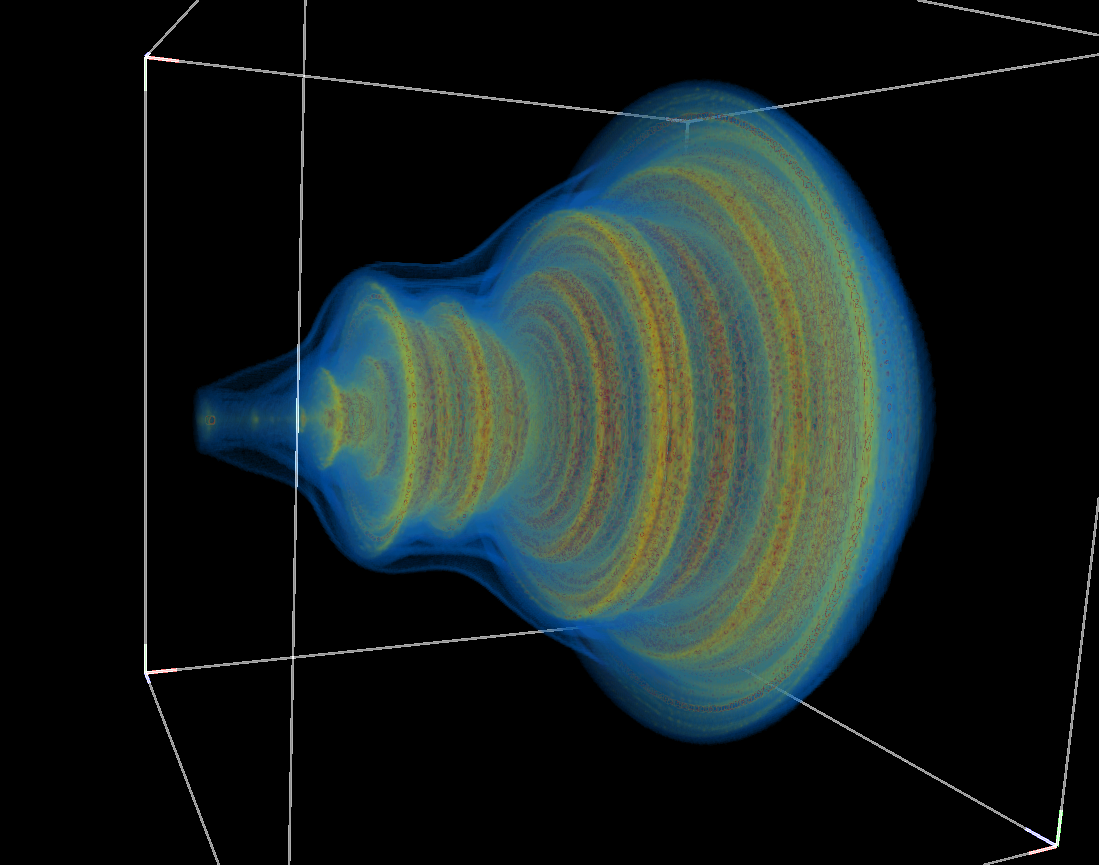# Quinternions

Attributed to Russell Walsmith
Written by Paul Bourke
August 2005

The images shown here are based upon quinternion arithmetic and are created in a similar way to the Mandelbrot set. That is, a function is iterated with some dependency on a position on the complex plane. The outcome of the series (infinity, zero, or otherwise) determines the state of that position and ultimately how it is represented graphically. Generally the distinction is made between the series tending to zero (inside the set) and other behaviour (outside the set).

The difference here is the function being iterated is not in the complex plane but rather in five dimensions. To determine whether the position in 5D is inside or outside the set, a test is made whether the magnitude of the series (a 5 dimensional vector) tends to zero. The result, as shown in the images on the right is a Mandelbrot like outline, extruded in 3D space over some limited range. In these image the first three dimensions are mapped to 3D Cartesian coordinates and the last two dimensions are fixed at one value, a point in the plane of the last two dimensions.

The series in five dimensions is given by
xn+1 = a + x0
yn+1 = b + y0
zn+1 = c + z0
un+1 = d + u0
vn+1 = e + v0
where
a = xn2 + 2 (ynvn + znun)
b = un2 + 2 (xnyn + znvn)
c = yn2 + 2 (xnzn + unvn)
d = vn2 + 2 (xnun + ynzn)
e = zn2 + 2 (xnvn + ynun)
where (x0,y0,z0,u0,v0) is the point in question.

C style code to evaluate the quinternion

```int Eval(double *q,int nmax)
{
int i,n=0;
double a,q1 = {0,0,0,0,0};
double zz = 0;

while (zz < 100 && n < nmax) {
a = q1*q1+2*(q1*q1+q1*q1);
a = q1*q1+2*(q1*q1+q1*q1);
a = q1*q1+2*(q1*q1+q1*q1);
a = q1*q1+2*(q1*q1+q1*q1);
a = q1*q1+2*(q1*q1+q1*q1);
zz = 0;
for (i=0;i<<5;i++) {
q1[i] = a[i] + q[i];
zz += q1[i] * q1[i];
}
n++;
}

return(n);
}
```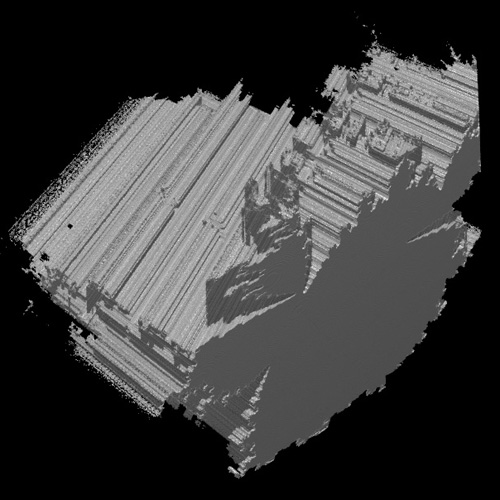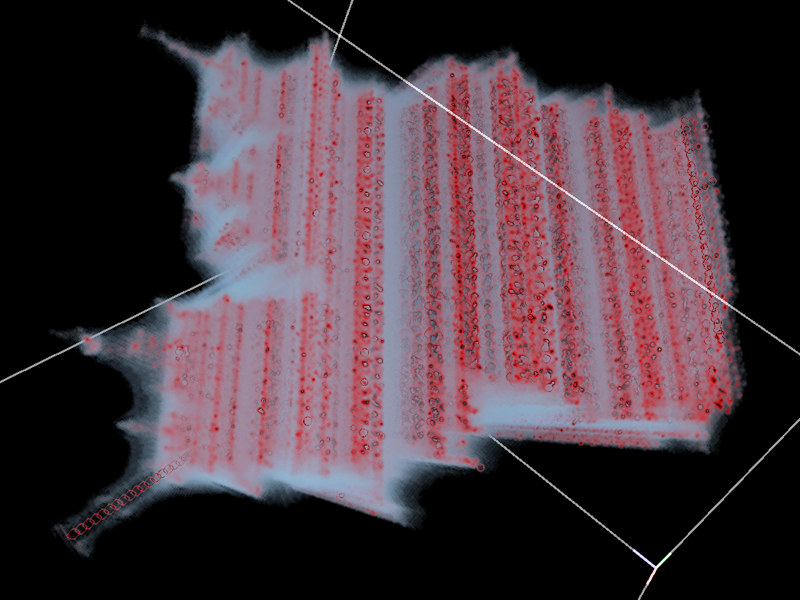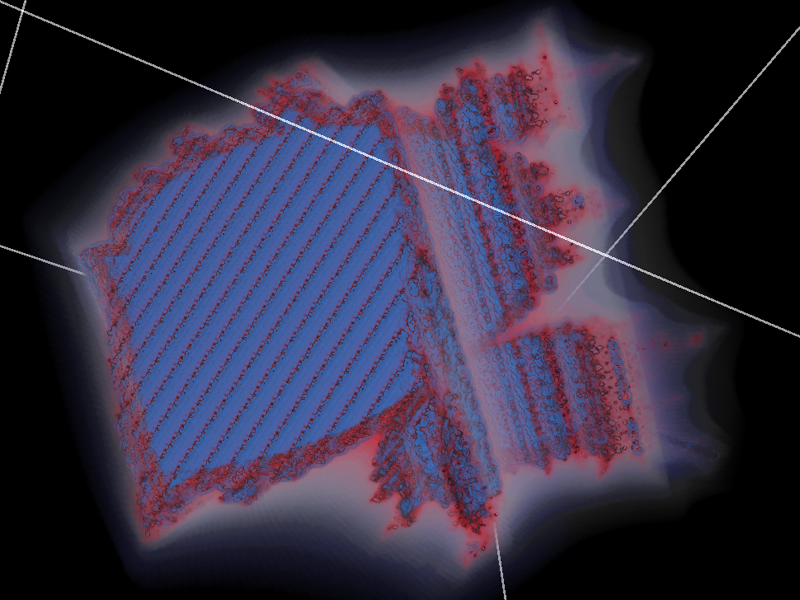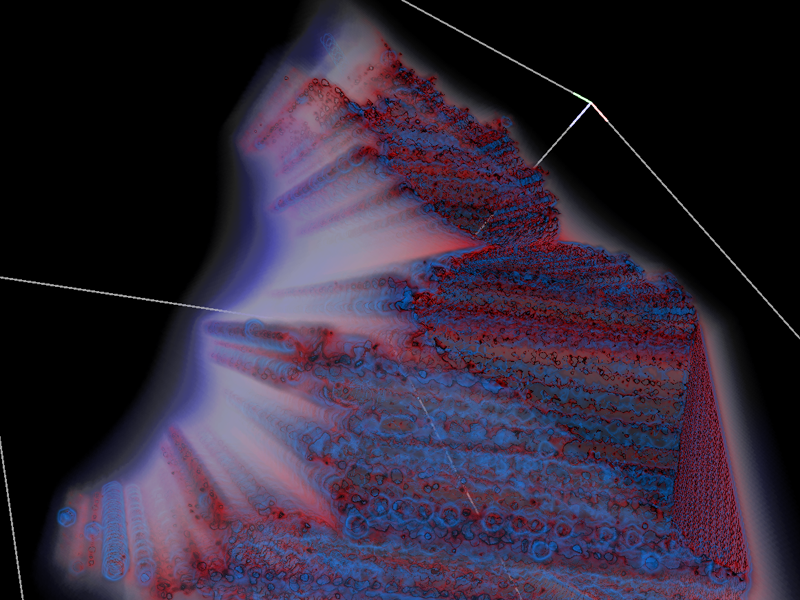# sine-mset

Created by Paul Bourke
Attributed to Russell Walsmith
January 2007

This is created by considering each point within some region of 3D space (x0,y0,z0) and evaluating the following series. The point (voxel in computer graphics language) is then shaded/coloured depending on how quickly the series tends to infinity.

xn+1 = a + sin(x0)
yn+1 = b + sin(y0)
zn+1 = c + sin(z0)

where

a = xn2 + 2 yn zn
b = zn2 + 2 xn yn
c = yn2 + 2 xn zn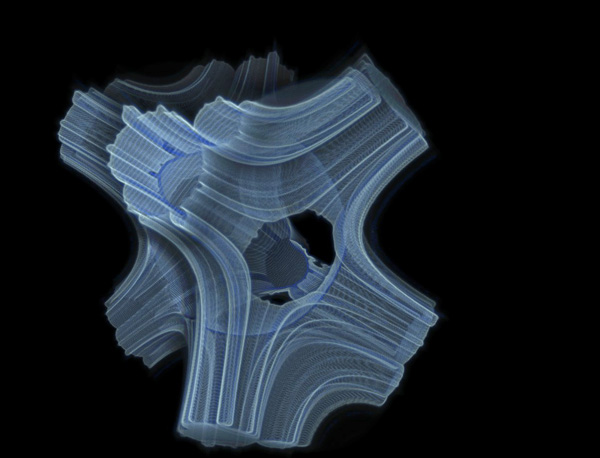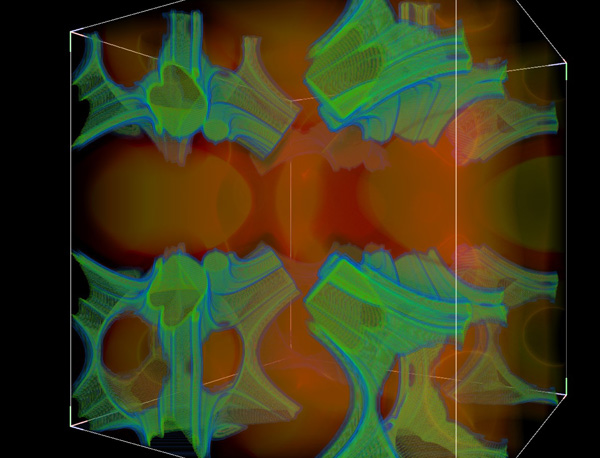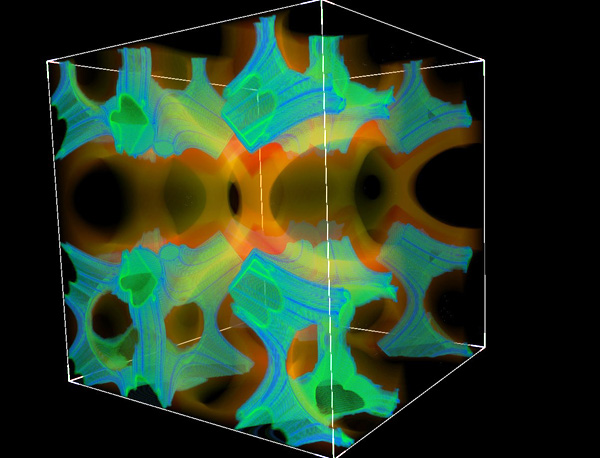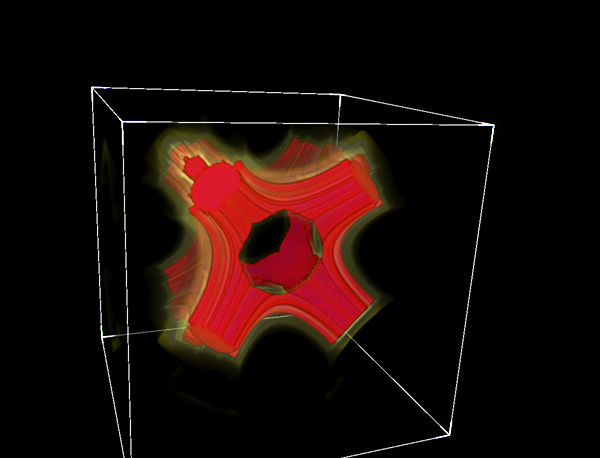# Triternions

Attributed to Russell Walsmith
Written by Paul Bourke
August 2005, Update: Oct 2014

The images shown here are based upon triternion arithmetic and are created in a similar way to the Mandelbrot set. That is, a function is iterated with some dependency on a position on the complex plane. The outcome of the series (infinity, zero, or otherwise) determines the state of that position and ultimately how it is represented graphically. Generally the distinction is made between the series tending to zero (inside the set) and other behaviour (outside the set).The difference here is the function being iterated is not in the complex plane but rather in three dimensions. To determine whether the position in 3D is inside or outside the set, a test is made whether the magnitude of the series (a vector) in 3D tends to zero. The result, as shown in the images is a Mandelbrot like outline, extruded in 3D space over some limited range.The series in three dimensions is given by
xn+1 = a + x0
yn+1 = b + y0
zn+1 = c + z0
where
a = xn2 + 2 yn zn
b = zn2 + 2 xn yn
c = yn2 + 2 xn zn
and (x0,y0,z0) is the point in question.In the images shown here the bounds of the cube shown is -1.75 to 0.75 in each dimension.

FracInt code by Russell Walsmith
```; The reduced Tset formula
Frm4 {
x=real(pixel), y=imag(pixel), z=p1
x1=y1=z1=0:
a = x1^2 + 2*y1*z1
b = z1^2 + 2*x1*y1
c = y1^2 + 2*x1*z1
x1 = a+x, y1 = b+y, z1 = c+z
s = x1^2 + y1^2 + v1^2
s < 100
}
```
C style code to evaluate the triternion
```int Eval(double x,double y,double z,int nmax)
{
int n = 0;
double x1 = 0,y1 = 0,z1 = 0;
double a,b,c;
double zz = 0;

while (zz < 100 && n < nmax) {
a = x1*x1 + 2*y1*z1;
b = z1*z1 + 2*x1*y1;
c = y1*y1 + 2*x1*z1;
x1 = a + x;
y1 = b + y;
z1 = c + z;
zz = x1*x1 + y1*y1 + z1*z1;
n++;
}

return(n);
}
```Update Oct 2014

Code to create a volume suitable for rendering with the Drishti volume visualisation software, although any volume rendering package could be used: triternion.c# Danca Fractal

Written by Paul Bourke
In conjunction with Marius-F Danca.
March 2008

The following images/visualisation are explorations of the series below, where z, c1, and c2 are all complex.

zn+1 = (zn2 + c1)2 + c2

Connectedness of Julia sets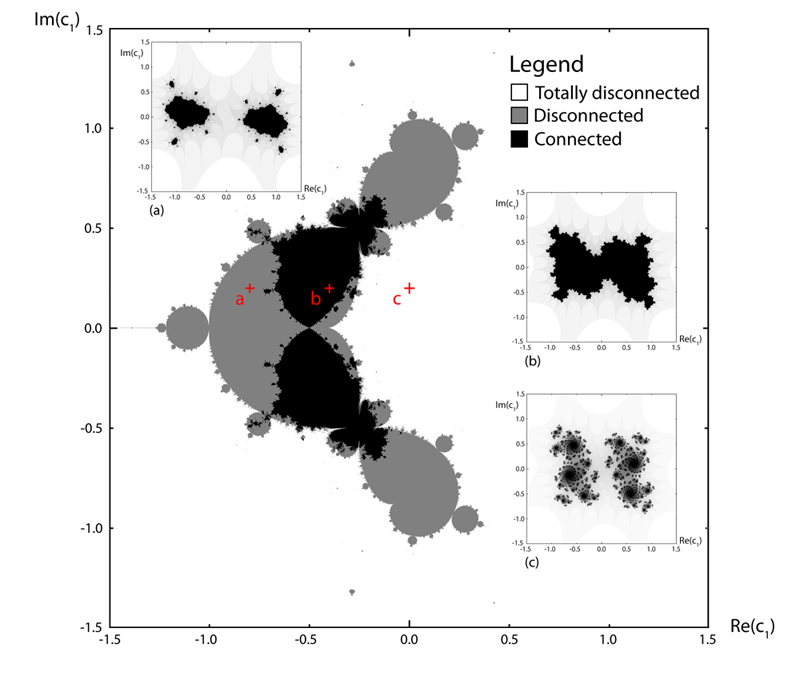3D forms resulting from slices of the 4D space by each axis plane (at the origin)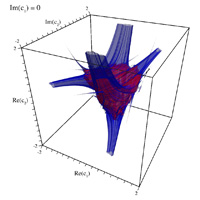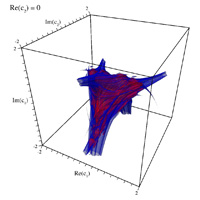Other images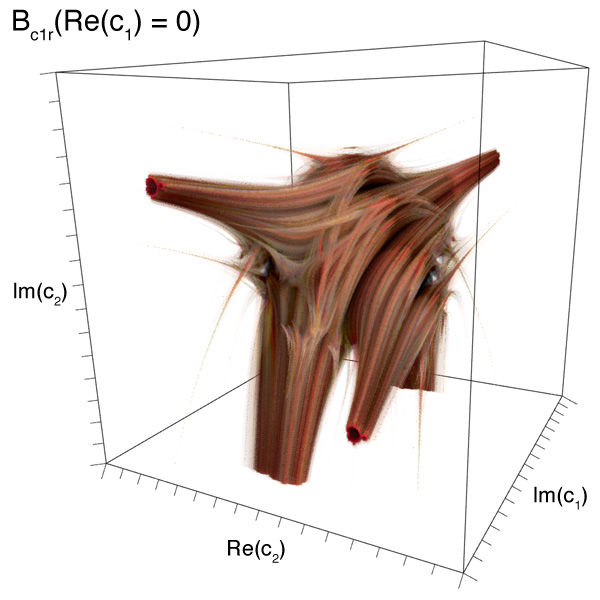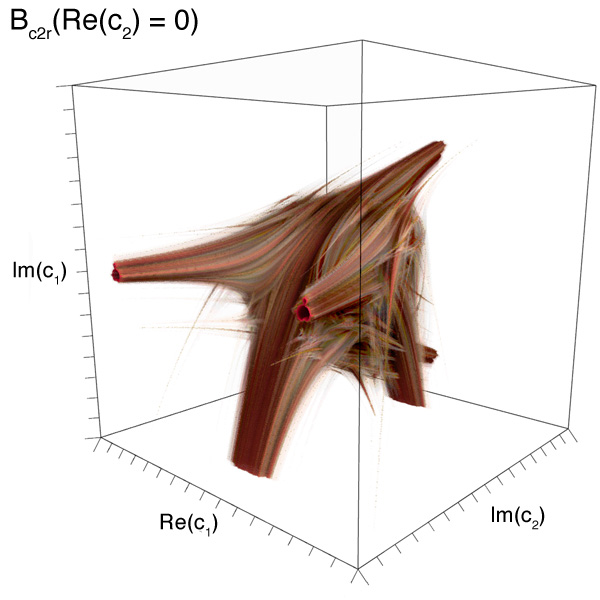Slices through 3D section